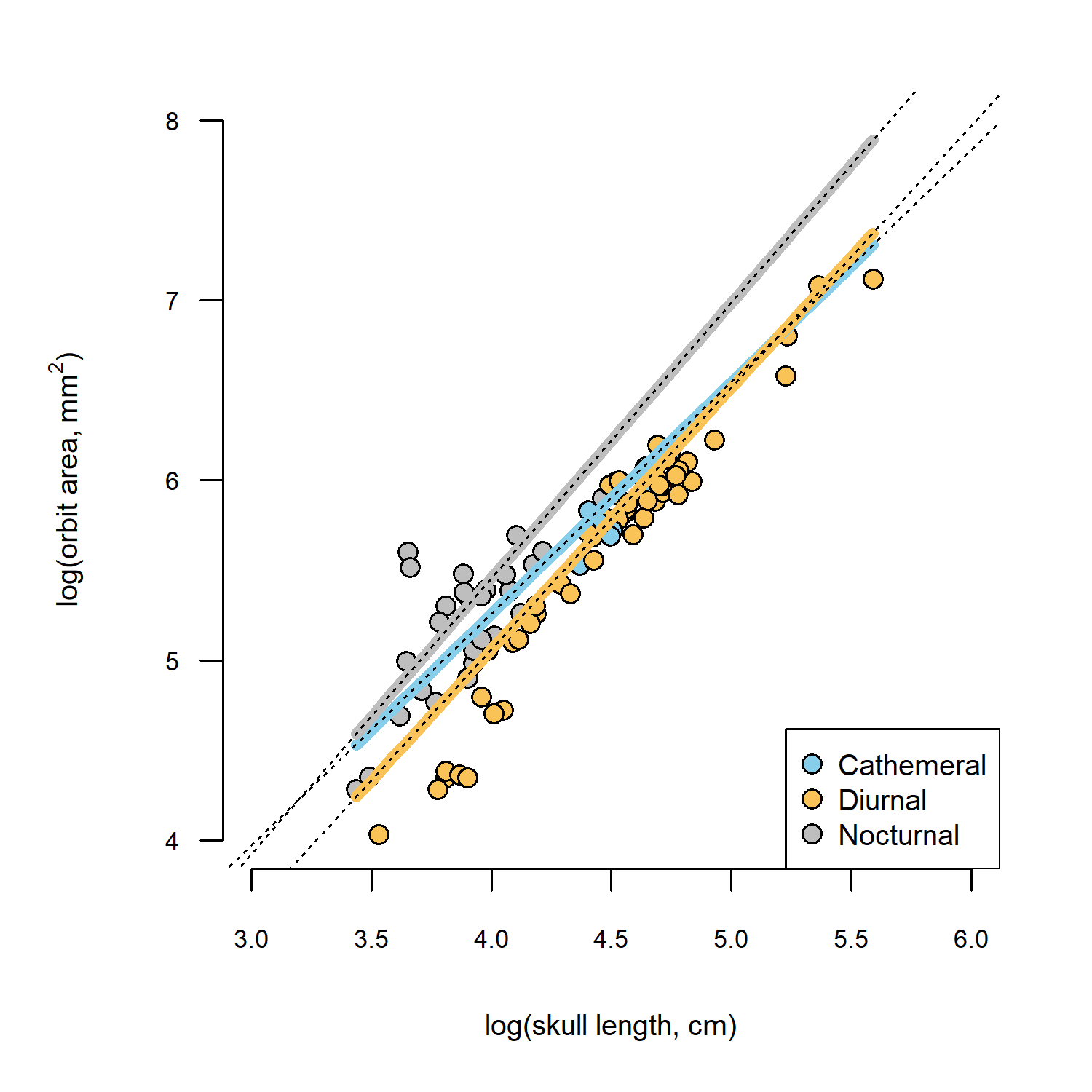## Tuesday, August 9, 2022

### Computing the slope & 95% CIs around the slope from a phylogenetic ANCOVA

“In the example in Chapter 3 where you analyze Rich Kay and Chris Kirk's data, I believe that I understand the output and that you also checked for interaction with activity pattern - no interaction and, therefore, similar slopes. The coefficients provide intercept differences between that of the cathemeral animals and those of the diurnal and nocturnal ones. These intercept differences make the calculation of the factor lines in Figure 3.3 possible if I'm understanding your walkthrough correctly. What I would like to know is whether it is possible to find the slopes and CIs for each group?”

This an excellent question & the answers are “yes” and “yes (but harder to plot them)”.

Let's see how.

To begin with, I'll load our packages and read our input data from file, here directly from the book website.

library(phytools)
library(nlme)
row.names=1,stringsAsFactors=TRUE)


Next, I'll build my hypothesized covariance structure of the residual error using corBrownian. If I had a different hypothesis, I could use the alternative function (e.g., corPagel) as appropriate.

spp<-rownames(primate.data)
corBM<-corBrownian(phy=primate.tree,form=~spp)


Now, I'm simply going to reiterate the analysis of Chapter 3 in the book, but this time allow different relationships between log(skull length) and log(orbit area). To do that, I write down the formula log(Orbit_area) ~ log(Skull_length) * Activity_pattern instead of log(Orbit_area) ~ log(Skull_length) + Activity_pattern from the book.

primate.ancova<-gls(log(Orbit_area)~log(Skull_length)*
Activity_pattern,data=primate.data,
correlation=corBM)


Here is a print-out of our model object.

primate.ancova

Generalized least squares fit by REML
Model: log(Orbit_area) ~ log(Skull_length) * Activity_pattern
Data: primate.data
Log-restricted-likelihood: 47.58855

Coefficients:
(Intercept)                           log(Skull_length)
0.1024294                                   1.2884912
Activity_patternDiurnal                   Activity_patternNocturnal
-0.8612406                                  -0.7781923
log(Skull_length):Activity_patternDiurnal log(Skull_length):Activity_patternNocturnal
0.1661815                                   0.2439095

Correlation Structure: corBrownian
Formula: ~spp
Parameter estimate(s):
numeric(0)
Degrees of freedom: 90 total; 84 residual
Residual standard error: 0.2960613


So, where's our slope for each factor level? Believe it or not, we can get this simply by adding up the corresponding coefficients.

So, for example, the intercept for the factor level Diurnal is the sum of (Intercept) and Activity_patternDiurnal; and the slope for factor level Diurnal is the sum of log(Skull_length) and log(Skull_length):Activity_patternDiurnal.

What about the coefficients for factor level Cathemeral? Well, just because this is our first factor level (alphabetically!) the intercept is (Intercept) and the slope is log(Skull_length): all the other slopes & intercepts are relative to those of our first factor level.

To illustrate this, I'm going to plot our data, our fitted ANCOVA model, from above (using predict, as we did in the book), and then finally, I'll add on our regression lines for each factor level using abline in which I set the intercept (a) and slope (b) as described above. Note that to do this I left our fitted model on the log-scale, rather than backtransforming to the original scale (as we did in the book).

par(mar=c(5.1,5.1,2.1,2.1),las=1)
pt.cols<-setNames(c("#87CEEB","#FAC358","grey"),
levels(primate.data$Activity_pattern)) plot(log(Orbit_area)~log(Skull_length), data=primate.data,pch=21, bg=pt.cols[primate.data$Activity_pattern],
bty="n",xlab="log(skull length, cm)",
ylab=expression(paste("log(orbit area, ",mm^2,")")),
cex=1.2,cex.axis=0.7,cex.lab=0.8,
xlim=c(3,6),ylim=c(4,8))
legend("bottomright",names(pt.cols),pch=21,pt.cex=1.2,
pt.bg=pt.cols,cex=0.8)
xx<-seq(min(primate.data$Skull_length), max(primate.data$Skull_length),length.out=100)
lines(log(xx),predict(primate.ancova,
newdata=data.frame(Skull_length=xx,
Activity_pattern=as.factor(rep("Cathemeral",100)))),
lwd=5,col=pt.cols["Cathemeral"])
lines(log(xx),predict(primate.ancova,
newdata=data.frame(Skull_length=xx,
Activity_pattern=as.factor(rep("Diurnal",100)))),
lwd=5,col=pt.cols["Diurnal"])
lines(log(xx),predict(primate.ancova,
newdata=data.frame(Skull_length=xx,
Activity_pattern=as.factor(rep("Nocturnal",100)))),
lwd=5,col=pt.cols["Nocturnal"])
## I'm adding the extra lines here
abline(a=coef(primate.ancova)["(Intercept)"],
b=coef(primate.ancova)["log(Skull_length)"],
lty="dotted")
abline(a=sum(coef(primate.ancova)[c("(Intercept)",
"Activity_patternDiurnal")]),
b=sum(coef(primate.ancova)[c("log(Skull_length)",
"log(Skull_length):Activity_patternDiurnal")]),
lty="dotted")
abline(a=sum(coef(primate.ancova)[c("(Intercept)",
"Activity_patternNocturnal")]),
b=sum(coef(primate.ancova)[c("log(Skull_length)",
"log(Skull_length):Activity_patternNocturnal")]),
lty="dotted")See how they line up exactly?

The second part of this question asked if it was possible to estimate confidence intervals around the regression slopes for each factor level.

This too is possible, although R certainly doesn't make it easy. (If readers know of a better solution than the one posted below, they should please share it and I will update this page.)

Our problems are twofold.

Firstly, the function predict for the "gls" object class does not include the standard options of interval="confidence" and "prediction" that we might know from the "lm" object.

Secondly, although we can use the function intervals to obtain confidence intervals on each parameter of our fitted model, this is not the same as confidence intervals on our individual factor slopes because these slopes are the sum of the coefficient for the factor level "Cathemeral" (in our case) and the interaction term for each factor level.

To estimate the CIs, then, for each slope, we must compute their values (as the sum), and then use the variance sum law to calculate the total variance of each estimate. The square root of this value multiplied by $$t_{d.f.}$$[α/2,1-α/2] should give us our confidence intervals.

df<-primate.ancova$dims$N-primate.ancova$dims$p
alpha<-0.05
ciBeta_Cathemeral<-coef(primate.ancova)["log(Skull_length)"]+
qt(c(alpha/2,1-alpha/2),df)*
sqrt(primate.ancova$varBeta[ "log(Skull_length)","log(Skull_length)"]) ciBeta_Cathemeral   0.5120424 2.0649401  ciBeta_Diurnal<-coef(primate.ancova)["log(Skull_length)"]+ coef(primate.ancova)["log(Skull_length):Activity_patternDiurnal"]+ qt(c(alpha/2,1-alpha/2),df)* sqrt(primate.ancova$varBeta[
"log(Skull_length)","log(Skull_length)"]+
primate.ancova$varBeta["log(Skull_length):Activity_patternDiurnal", "log(Skull_length):Activity_patternDiurnal"]+ 2*primate.ancova$varBeta["log(Skull_length)",
"log(Skull_length):Activity_patternDiurnal"])
ciBeta_Nocturnal<-coef(primate.ancova)["log(Skull_length)"]+
coef(primate.ancova)["log(Skull_length):Activity_patternNocturnal"]+
qt(c(alpha/2,1-alpha/2),df)*
sqrt(primate.ancova$varBeta[ "log(Skull_length)","log(Skull_length)"]+ primate.ancova$varBeta["log(Skull_length):Activity_patternNocturnal",
"log(Skull_length):Activity_patternNocturnal"]+
2*primate.ancova$varBeta["log(Skull_length)", "log(Skull_length):Activity_patternNocturnal"])  CIs<-data.frame(parameter= paste("beta(",levels(primate.data$Activity_pattern),")",sep=""),
lower=c(ciBeta_Cathemeral,ciBeta_Diurnal,ciBeta_Nocturnal),
estimate=coef(primate.ancova)["log(Skull_length)"]+c(0,
coef(primate.ancova)[c("log(Skull_length):Activity_patternDiurnal",
"log(Skull_length):Activity_patternNocturnal")]),
upper=c(ciBeta_Cathemeral,ciBeta_Diurnal,ciBeta_Nocturnal))
rownames(CIs)<-NULL
CIs

         parameter     lower estimate    upper
1 beta(Cathemeral) 0.5120424 1.288491 2.064940
2    beta(Diurnal) 1.2729517 1.454673 1.636394
3  beta(Nocturnal) 1.2367092 1.532401 1.828092


From this table, we see that the confidence intervals for the slopes of our three different factor levels are overlapping.

We can doublecheck our interval for the factor level "Cathemeral" only by using intervals and comparing it to "log(Skull_length)".

intervals(primate.ancova)

Approximate 95% confidence intervals

Coefficients:
lower       est.     upper
(Intercept)                                 -3.4299976  0.1024294 3.6348563
log(Skull_length)                            0.5120424  1.2884912 2.0649401
Activity_patternDiurnal                     -4.4138559 -0.8612406 2.6913746
Activity_patternNocturnal                   -4.4919422 -0.7781923 2.9355577
log(Skull_length):Activity_patternDiurnal   -0.6170616  0.1661815 0.9494247
log(Skull_length):Activity_patternNocturnal -0.5861534  0.2439095 1.0739724

Residual standard error:
lower      est.     upper
0.2572684 0.2960613 0.3487392


We see that they match.

A kind of clunky way to do the same for each of the factor levels is as follows. What I'll do is reorder the levels of our factor so that (instead of "Cathemeral") "Diurnal" is the first factor level, and then "Nocturnal".

primate.data2<-primate.data
primate.data2$Activity_pattern<-factor( as.character(primate.data$Activity_pattern),
levels(primate.data$Activity_pattern)[c(2,1,3)]) intervals(gls(log(Orbit_area)~log(Skull_length)* Activity_pattern,data=primate.data2, correlation=corBM))$coef[2,,drop=FALSE]

                     lower     est.    upper
log(Skull_length) 1.272952 1.454673 1.636394

primate.data3<-primate.data
primate.data3$Activity_pattern<-factor( as.character(primate.data$Activity_pattern),
levels(primate.data$Activity_pattern)[c(3,1,2)]) intervals(gls(log(Orbit_area)~log(Skull_length)* Activity_pattern,data=primate.data3, correlation=corBM))$coef[2,,drop=FALSE]

                     lower     est.    upper
log(Skull_length) 1.236709 1.532401 1.828092


We see that they match our prior estimates exactly!